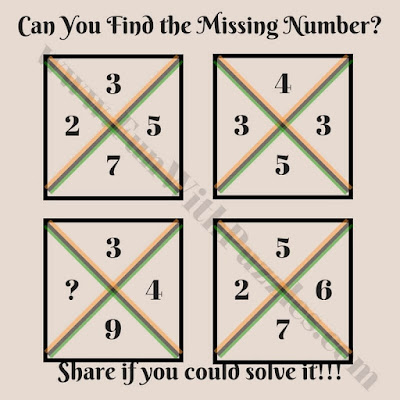How good are you in Mathematics? Here is time to test your Math skills. In this mind-blowing Math Question, there are four numbers in rectangles. These numbers form some logical or mathematical pattern. Your challenge is to find this pattern and then find the missing number which replaces questions mark. Can you find this missing number?Can you find the missing number?

Victor Etim said...

How

Explain better

Fun With Puzzles said...

Starting from top number and moving clockwise, lets mark the given numbers in each Rectangle as A, B, C and D. The Logical Reasoning in this Mind Blowing Math Question is as below
A+C=B*D
Please do let us know if anything is still not clear?

Unknown said...